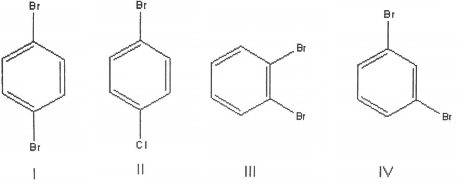# Problem: What is the correct assignment of the number of signals in the  13C NMR spectra of the following compounds? a. I=2 sig., II=4 sig., III=3 sig., IV=4 sig. b. I=3 sig., II=4 sig., III=4 sig., IV=5 sig. c. I=2 sig., II=2 sig., III=3 sig., IV=4 sig. d. I=2 sig., II=4 sig., III=5 sig., IV=6 sig. e. I=2 sig., II=3 sig., III=4 sig., IV=5 sig.

🤓 Based on our data, we think this question is relevant for Professor Frazer's class at UCF.

###### Problem Details

What is the correct assignment of the number of signals in the  13C NMR spectra of the following compounds?

a. I=2 sig., II=4 sig., III=3 sig., IV=4 sig.
b. I=3 sig., II=4 sig., III=4 sig., IV=5 sig.
c. I=2 sig., II=2 sig., III=3 sig., IV=4 sig.
d. I=2 sig., II=4 sig., III=5 sig., IV=6 sig.
e. I=2 sig., II=3 sig., III=4 sig., IV=5 sig.What scientific concept do you need to know in order to solve this problem?

Our tutors have indicated that to solve this problem you will need to apply the 13C NMR: Cumulative Practice concept. If you need more 13C NMR: Cumulative Practice practice, you can also practice 13C NMR: Cumulative Practice practice problems.

What is the difficulty of this problem?

Our tutors rated the difficulty ofWhat is the correct assignment of the number of signals in t...as high difficulty.

How long does this problem take to solve?

Our expert Organic tutor, Chris took 4 minutes and 10 seconds to solve this problem. You can follow their steps in the video explanation above.

What professor is this problem relevant for?

Based on our data, we think this problem is relevant for Professor Frazer's class at UCF.